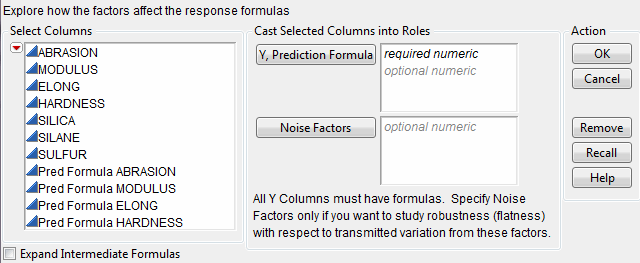Publication date: 08/13/2020

## Profiler Launch Windows

When a profiler is invoked as a platform from the Graph menu, rather than through a fitting platform, you provide columns with formulas as the Y, Prediction Formula columns. These formulas could have been saved from the fitting platforms.

Figure 2.3 Profiler Launch WindowThe columns referenced in the formulas become the X columns (unless the column is also a Y).

Y, Prediction Formula

The response columns containing formulas.

Noise Factors

Used only in special cases for modeling derivatives. For more information about noise factors, see Noise Factors.

Expand Intermediate Formulas

Tells JMP that if an ingredient column to a formula is itself a formula column that refers to other columns, to substitute the original columns into the inner formula. To prevent an ingredient column from expanding, add an Other column property, name it Expand Formula, and assign a value of 0. See Expand Intermediate Formulas.

The Surface Plot platform is discussed in Surface Plot. The Surface Profiler is very similar to the Surface Plot platform, except Surface Plot has more modes of operation. Neither the Surface Plot platform nor the Surface Profiler have some of the capabilities common to other profilers.

### Expand Intermediate Formulas

The Profiler launch window has an Expand Intermediate Formulas check box. When this box is checked, the formula that is being profiled is handled differently. If the profiled formula contains another formula column that references other columns, then the original columns are substituted into the inner formula. Therefore, the formula being profiled is profiled with respect to the original columns instead of the intermediate column references. Expand Intermediate Formula also expands formula columns that have the Vector modeling type.

For example, when Fit Model fits a logistic regression for two levels (A and B), the end formulas (Prob[A] and Prob[B]) are functions of the Lin[x] column, which itself is a function of another column x. If Expand Intermediate Formulas is selected, then when Prob[A] is profiled, it is with reference to x, not Lin[x].

In addition, using the Expand Intermediate Formulas check box enables the Save Expanded Formulas command in the platform red triangle menu. This creates a new column with a formula, which is the formula being profiled as a function of the end columns, not the intermediate columns.